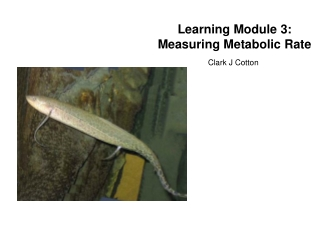DownloadDownload PresentationLearning Module 3: Measuring Metabolic Rate

# Learning Module 3: Measuring Metabolic Rate

Télécharger la présentation## Learning Module 3: Measuring Metabolic Rate

- - - - - - - - - - - - - - - - - - - - - - - - - - - E N D - - - - - - - - - - - - - - - - - - - - - - - - - - -
##### Presentation Transcript

1. Learning Module 3:Measuring Metabolic Rate Clark J Cotton

2. Measuring Metabolic Rate Metabolic Rate: the sum of all energetic processes occuring in the body.

3. Measuring Metabolic Rate Metabolic Rate: the sum of all energetic processes occuring in the body. How do you measure metabolic rate?

4. Measuring Metabolic Rate Metabolic Rate: the sum of all energetic processes occuring in the body. How do you measure metabolic rate? • Direct Calorimetry

5. Measuring Metabolic Rate Metabolic Rate: the sum of all energetic processes occuring in the body. How do you measure metabolic rate? • Direct Calorimetry • Mass Balance

6. Measuring Metabolic Rate Metabolic Rate: the sum of all energetic processes occuring in the body. How do you measure metabolic rate? • Direct Calorimetry • Mass Balance • Oxygen Consumption

7. What’s Oxygen Got to do with It? • Oxygen is the basic reducing compound used to convert sugar to carbon dioxide, releasing energy for our bodies and producing carbon dioxide. • Depending on level of activity, almost all of our metabolism is dependent on oxygen. 6 O2 + C6H12O6 6 CO2 + 6 H2O

8. Measuring Metabolic Rate • Materials: 1 empty milk jug, plastic tubing, 500ml beaker, permanent markers. • Gradually fill up milk jug in 500ml increments, mark each addition. • Flip jug upside down in tub of water and insert plastic tube. • If you breath in through your nose, and out through your mouth you can calculate the amount of air you breath.

9. Tidal Volumes • Tidal volume is the volume of air you inhale and then exhale with each breath.

10. Vital Capacity • Vital Capacity is the maximum amount of air that you can inhale and exhale.

11. Dead Space • Dead space volume is the volume of air that does not participate in gas exchange because of airway volume. Dead space volume (ml) = body weight (kg) * 2.2

12. Total Lung Capacity • Total lung capacity is equal to the vital capacity + the dead space.

13. If you know the number of breaths you take per minute, and the average volume of air per breath, you can calculate the total volume of air you’re breathing per hour. Assuming that the air you breath contains 21% oxygen, and the air you exhale contains 17% oxygen, you can calculate your total oxygen consumption per hour. Example: 15 breaths / minute 500ml air / breath 450 L air / hour (.21*450) – (.17*450) = 18 L O2 per hour Measuring Metabolic Rate

14. Possibilities with Metabolic Rate Measurements Effects of: • Age • Exercise • Size • Temperature • Time of Day

15. Possibilities with Metabolic Rate Measurements Effects of: • Age • Exercise • Size • Temperature • Time of Day

16. Possibilities with Metabolic Rate Measurements Effects of: • Age • Exercise • Size • Temperature • Time of Day

17. Possibilities with Metabolic Rate Measurements Effects of: • Age • Exercise • Size • Temperature • Time of Day

18. Possibilities with Metabolic Rate Measurements Effects of: • Age • Exercise • Size • Temperature • Time of Day